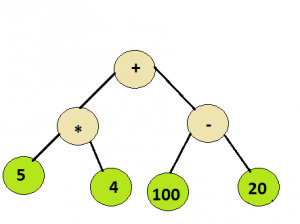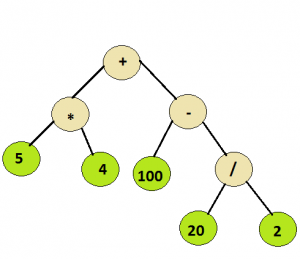# Evaluation of Expression Tree

Given a simple expression tree, consisting of basic binary operators i.e., + , – ,* and / and some integers, evaluate the expression tree.

Examples:

```
Input :
Root node of the below treeOutput :
100

Input :
Root node of the below treeOutput :
110
```

## Recommended: Please solve it on “PRACTICE” first, before moving on to the solution.

As all the operators in the tree are binary hence each node will have either 0 or 2 children. As it can be inferred from the examples above , the integer values would appear at the leaf nodes , while the interior nodes represent the operators.
To evaluate the syntax tree , a recursive approach can be followed .

```Algorithm :
Let t be the syntax tree
If  t is not null then
If t.info is operand then
Return  t.info
Else
A = solve(t.left)
B = solve(t.right)
return A operator B
where operator is the info contained in t

```

The time complexity would be O(n), as each node is visited once. Below is a C++ program for the same:

## C/C++

 `// C++ program to evaluate an expression tree  ` `#include   ` `using` `namespace` `std;  ` ` `  `// Class to represent the nodes of syntax tree  ` `class` `node  ` `{  ` `public``:  ` `    ``string info;  ` `    ``node *left = NULL, *right = NULL;  ` `    ``node(string x)  ` `    ``{  ` `        ``info = x;  ` `    ``}  ` `};  ` ` `  `// Utility function to return the integer value  ` `// of a given string  ` `int` `toInt(string s)  ` `{  ` `    ``int` `num = 0;  ` `       `  `      ``// Check if the integral value is  ` `      ``// negative or not ` `      ``// If it is not negative, generate the number  ` `      ``// normally ` `      ``if``(s!=``'-'``) ` `        ``for` `(``int` `i=0; ileft && !root->right)  ` `        ``return` `toInt(root->info);  ` ` `  `    ``// Evaluate left subtree  ` `    ``int` `l_val = eval(root->left);  ` ` `  `    ``// Evaluate right subtree  ` `    ``int` `r_val = eval(root->right);  ` ` `  `    ``// Check which operator to apply  ` `    ``if` `(root->info==``"+"``)  ` `        ``return` `l_val+r_val;  ` ` `  `    ``if` `(root->info==``"-"``)  ` `        ``return` `l_val-r_val;  ` ` `  `    ``if` `(root->info==``"*"``)  ` `        ``return` `l_val*r_val;  ` ` `  `    ``return` `l_val/r_val;  ` `}  ` ` `  `//driver function to check the above program  ` `int` `main()  ` `{  ` `    ``// create a syntax tree  ` `    ``node *root = ``new` `node(``"+"``);  ` `    ``root->left = ``new` `node(``"*"``);  ` `    ``root->left->left = ``new` `node(``"5"``);  ` `    ``root->left->right = ``new` `node(``"-4"``);  ` `    ``root->right = ``new` `node(``"-"``);  ` `    ``root->right->left = ``new` `node(``"100"``);  ` `    ``root->right->right = ``new` `node(``"20"``);  ` `    ``cout << eval(root) << endl;  ` ` `  `    ``delete``(root);  ` ` `  `    ``root = ``new` `node(``"+"``);  ` `    ``root->left = ``new` `node(``"*"``);  ` `    ``root->left->left = ``new` `node(``"5"``);  ` `    ``root->left->right = ``new` `node(``"4"``);  ` `    ``root->right = ``new` `node(``"-"``);  ` `    ``root->right->left = ``new` `node(``"100"``);  ` `    ``root->right->right = ``new` `node(``"/"``);  ` `    ``root->right->right->left = ``new` `node(``"20"``);  ` `    ``root->right->right->right = ``new` `node(``"2"``);  ` ` `  `    ``cout << eval(root);  ` `    ``return` `0;  ` `}  `

## Python

 `# Python program to evaluate expression tree ` ` `  `# Class to represent the nodes of syntax tree ` `class` `node: ` `    ``def` `__init__(``self``, value): ` `        ``self``.left ``=` `None` `        ``self``.data ``=` `value ` `        ``self``.right ``=` `None` ` `  `# This function receives a node of the syntax tree ` `# and recursively evaluate it ` `def` `evaluateExpressionTree(root): ` ` `  `    ``# empty tree ` `    ``if` `root ``is` `None``: ` `        ``return` `0` ` `  `    ``# leaf node ` `    ``if` `root.left ``is` `None` `and` `root.right ``is` `None``: ` `        ``return` `int``(root.data) ` ` `  `    ``# evaluate left tree ` `    ``left_sum ``=` `evaluateExpressionTree(root.left) ` ` `  `    ``# evaluate right tree ` `    ``right_sum ``=` `evaluateExpressionTree(root.right) ` ` `  `    ``# check which operation to apply ` `    ``if` `root.data ``=``=` `'+'``: ` `        ``return` `left_sum ``+` `right_sum ` `     `  `    ``elif` `root.data ``=``=` `'-'``: ` `        ``return` `left_sum ``-` `right_sum ` `     `  `    ``elif` `root.data ``=``=` `'*'``: ` `        ``return` `left_sum ``*` `right_sum ` `     `  `    ``else``: ` `        ``return` `left_sum ``/` `right_sum ` ` `  `# Driver function to test above problem ` `if` `__name__``=``=``'__main__'``: ` `     `  `    ``# creating a sample tree ` `    ``root ``=` `node(``'+'``) ` `    ``root.left ``=` `node(``'*'``) ` `    ``root.left.left ``=` `node(``'5'``) ` `    ``root.left.right ``=` `node(``'4'``) ` `    ``root.right ``=` `node(``'-'``) ` `    ``root.right.left ``=` `node(``'100'``) ` `    ``root.right.right ``=` `node(``'20'``) ` `    ``print` `evaluateExpressionTree(root) ` ` `  `    ``root ``=` `None` ` `  `    ``#creating a sample tree ` `    ``root ``=` `node(``'+'``) ` `    ``root.left ``=` `node(``'*'``) ` `    ``root.left.left ``=` `node(``'5'``) ` `    ``root.left.right ``=` `node(``'4'``) ` `    ``root.right ``=` `node(``'-'``) ` `    ``root.right.left ``=` `node(``'100'``) ` `    ``root.right.right ``=` `node(``'/'``) ` `    ``root.right.right.left ``=` `node(``'20'``) ` `    ``root.right.right.right ``=` `node(``'2'``) ` `    ``print` `evaluateExpressionTree(root) ` ` `  `# This code is contributed by Harshit Sidhwa `

Output:

```100
110
```

This article is contributed by Ashutosh Kumar. If you like GeeksforGeeks and would like to contribute, you can also write an article using contribute.geeksforgeeks.org or mail your article to contribute@geeksforgeeks.org. See your article appearing on the GeeksforGeeks main page and help other Geeks.